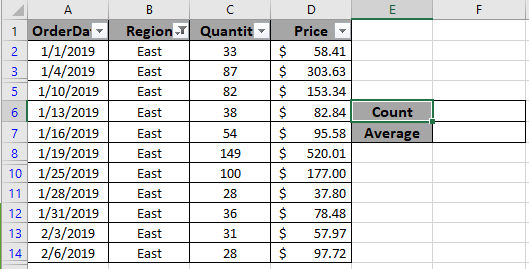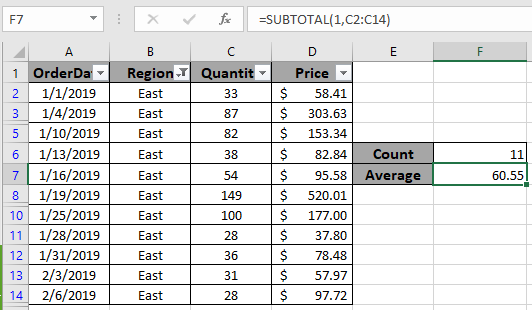# Excel Count filtered row using SUBTOTAL function

In this article, we will learn how to Count visible filtered rows.

Scenario:

In simple words, while working with table values, sometimes we need to count, sum, product or average of the values having already applied filter option. Example if we need to get the count of a certain IDs where the ID data has applied filter on. Criteria on data is applied using the filter option.

How to solve the problem ?

For this article we will be required to use the SUBTOTAL function. Now we will make a formula out of the function. Here we are given some values in a range and specific text value as criteria. We need to count the values where the formula includes all the values which ends with the given text or pattern

Generic formula:-

= SUBTOTAL ( fun_num, range )

fun_num : number, num corresponding to the operation

range : range where operation is to apply.

Example:

All of these might be confusing to understand. So, let's test this formula via running it on the example shown below.

Here we have the order import data. And we have applied criteria to Region, only the East region is filtered.Here to find the count of filtered values. Choose the right argument as fun_num. Fun_num is the operation you want to apply. Here to count the cells we use COUNTA operation, num as 3.
Use the formula:

= SUBTOTAL ( 3, B2:B14)As you can see the total rows which are visible comes out to be 11. Now we will use one more example to extract the Average of the quantity which are visible or filtered.

Use the formula:

= SUBTOTAL ( 1 , C2:C14 )As you can see the average order quantity received from the East region comes out to be approx 60. You can use different operations like average, count, max, min, product, standard deviation, sum or variation as per the required results on filtered data.

Here are some observational notes shown below.

Notes:

1. Choose the right fun_num to get the desired results.
2. The formula only works with numbers and text both
3. Operators like equals to ( = ), less than equal to ( <= ), greater than ( > ) or not equals to ( <> ) can be performed within function applied on numbers only.

Hope this article about how to Count visible filtered rows in Excel is explanatory. Find more articles on COUNTIFS functions here. If you liked our blogs, share it with your friends on Facebook. And also you can follow us on Twitter and Facebook. We would love to hear from you, do let us know how we can improve, complement or innovate our work and make it better for you. Write us at info@exceltip.com

Related Articles

How to use the SUBTOTAL function in Excel: Returns the SUM, COUNT, AVERAGE, STDEV or PRODUCT on applied filtered data in Excel.

COUNTIFS with Dynamic Criteria Range : Count cells dependent on other cell values in Excel.

COUNTIFS Two Criteria Match : Count cells matching two different criteria on list in excel.

COUNTIFS With OR For Multiple Criteria : Count cells having multiple criteria match using the OR function.

The COUNTIFS Function in Excel : Count cells dependent on other cell values.

How to Use Countif in VBA in Microsoft Excel : Count cells using Visual Basic for Applications code.

How to use wildcards in excel : Count cells matching phrases using the wildcards in excel

Popular Articles

50 Excel Shortcut to Increase Your Productivity

Edit a dropdown list

Absolute reference in Excel

If with conditional formatting

If with wildcards

Vlookup by date

Terms and Conditions of use

The applications/code on this site are distributed as is and without warranties or liability. In no event shall the owner of the copyrights, or the authors of the applications/code be liable for any loss of profit, any problems or any damage resulting from the use or evaluation of the applications/code.